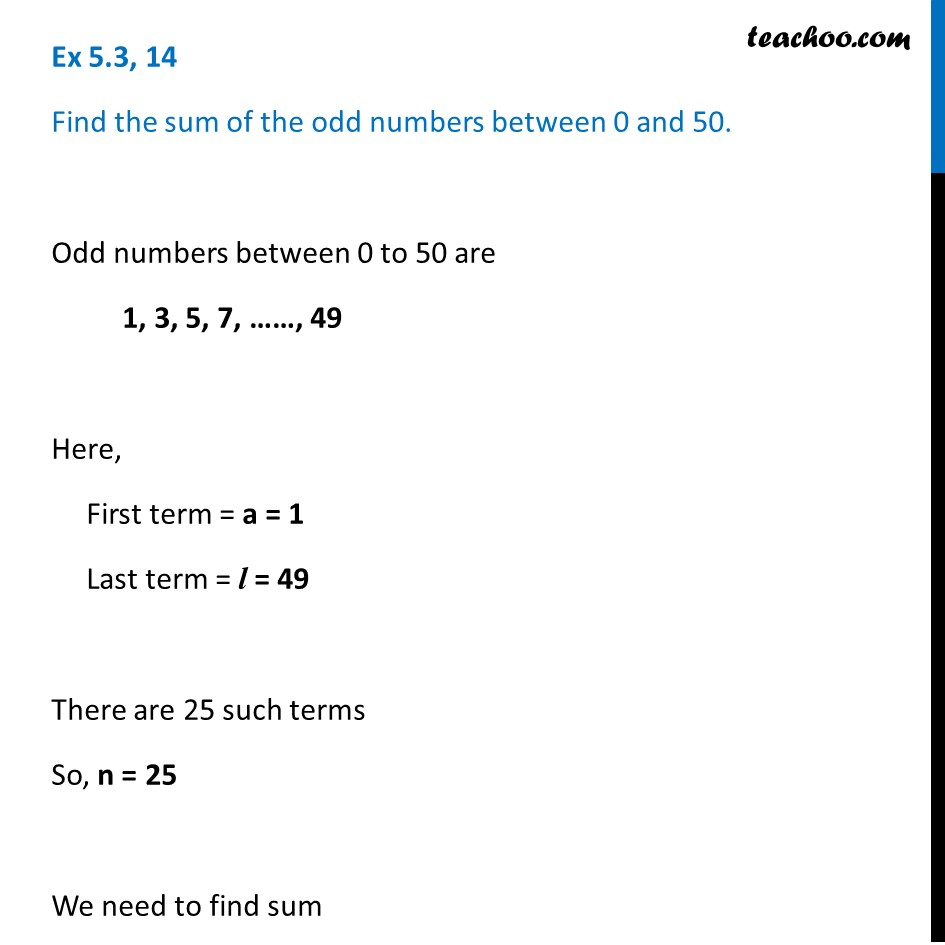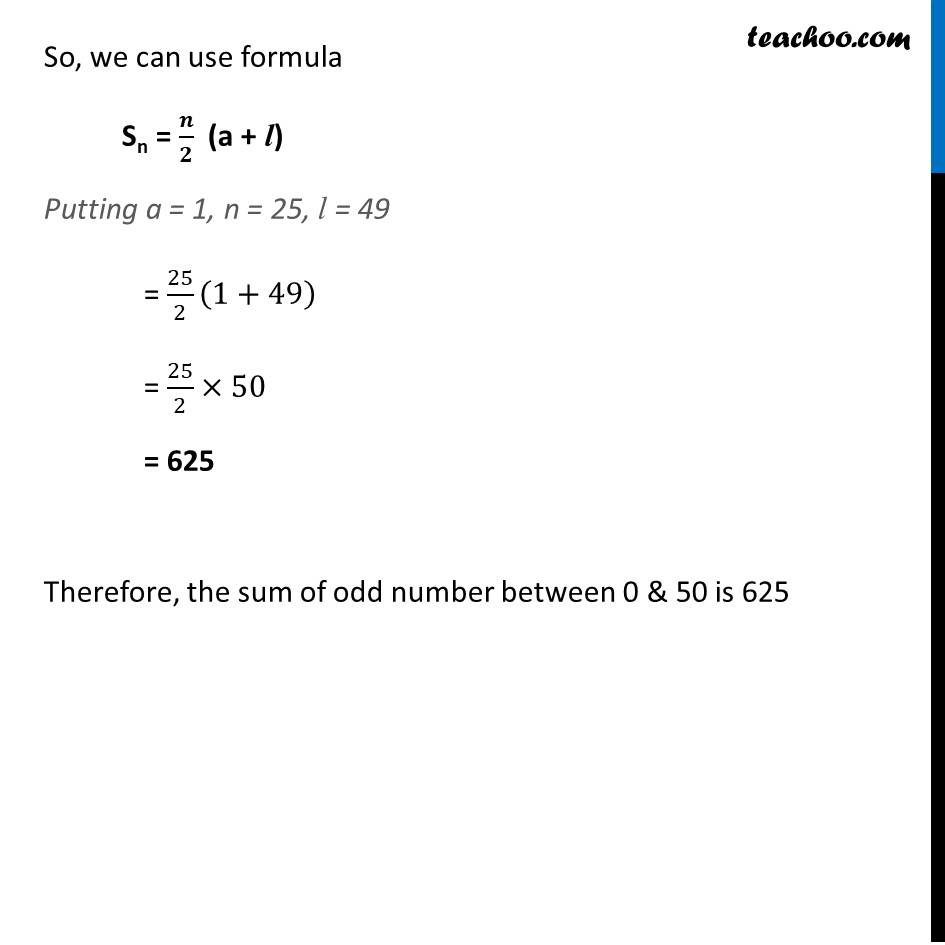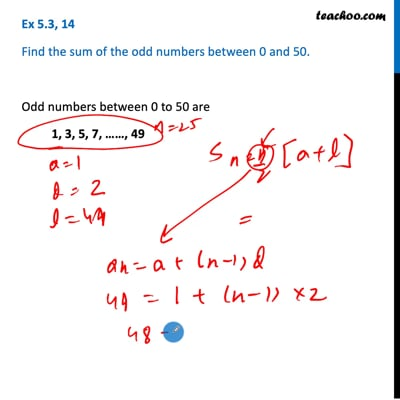Ex 5.3

Chapter 5 Class 10 Arithmetic Progressions
Serial order wiseThis video is only available for Teachoo black users

Solve all your doubts with Teachoo Black (new monthly pack available now!)

### Transcript

Ex 5.3, 14 Find the sum of the odd numbers between 0 and 50. Odd numbers between 0 to 50 are 1, 3, 5, 7, ……, 49 Here, First term = a = 1 Last term = l = 49 There are 25 such terms So, n = 25 We need to find sum So, we can use formula Sn = 𝒏/𝟐 (a + l) Putting a = 1, n = 25, l = 49 = 25/2 (1+49) = 25/2×50 = 625 Therefore, the sum of odd number between 0 & 50 is 625